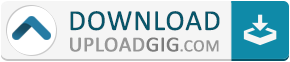#Do not remember me!

### Categories» » » Egghead - Introductory Machine Learning Algorithms in Python with scikit-learn

## Egghead - Introductory Machine Learning Algorithms in Python with scikit-learnType Tutorials
Language English
Total size 84.3 MB

Artificial intelligence. Machine learning. Bots. Computers learning and communicating with us to do our bidding. But, where do you start? How do you get a machine to even begin to understand what you speak or type at it? There are several common machine learning algorithms that will help us begin to answer these questions.

In this course we’ll learn about common machine learning algorithms that don’t require implementing a neural network. We will not be going too much into the math behind them, but instead learn what each algorithm is good for, and how to train them. We'll also learn about a few metrics for evaluating models.

We’ll implement these in Python using scikit-learn using scikit-learn’s built-in data sets. The focus of this course is on implementation and a high-level understanding of these algorithms.

We'll look at a few ways to evaluate our models, for both classification and regression models. We'll touch on mean squared error and coefficient of determination (for regression), and accuracy score, logarithmic loss, confusion matrices, and classification reports (for classification).

Python 2.7 is used in the lesson videos but the code provided has Python 3 available. The only breaking change is the print statement API.Name:* E-Mail: Security Code: *